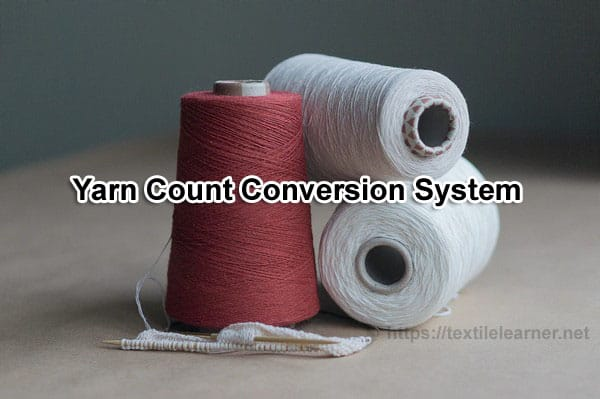# Count Conversion System for Textile Yarns

Last Updated on 17/01/2021

### Count Conversion System for Textile Yarns

Rana Sohel
Executive Engineer
Sunman Textile Mills Ltd. Chittagong
Email: rsohel07tex@gmail.com

Introduction:
To get all the information about textile yarn count I write this article. It will help the spinners to know all information about yarn count in one place at a glance. Before going to count conversion system, at first we should to know terms. Here count related terms are given below.

Yarn Count:
Count is a numerical value, which express the coarseness or fineness (diameter) of the yarn and also indicate the relationship between length and weight (the mass per unit length or the length per unit mass) of that yarn. Yarn count is also called yarn number.There are two systems of yarn count or numbering:

1. Direct system
2. Indirect system

In direct system, yarn number is called the linear density of yarn with units of tex, denier and dtex, etc. Similarly, in the indirect system yarn number is called the yarn count with units of NEC, Nm and N woollen, etc.

Following is the detailed explanation of these two systems:

A. Indirect system
It is used for the measurement of length per unit weight of the yarn. In this system, weight is kept constant while length is variable.

In the indirect system, yarn thickness and yarn number are inversely proportional. This means that as the yarn count increases the yarn weight decreases and hence yarn becomes finer.

The indirect system is also known as English system of counting. The most commonly used indirect numbering systems are

……………………………………..No of hanks
1. English Cotton, Ne = ————————–
…………………………………………..lbs

Ne: The English cotton count indicates the weight in lb of material of 840 yds length.

………………………………………..Km
2. Metric system, Nm = —————-
………………………………………..Kg

Nm: The metric yarn count indicates how many Km of yarn are contained in 1 kg or how many meters of yarn are contained in 1 gram.

B. Direct system
It is used for the measurement of linear density that is the weight per unit length of yarn. In this system yarn length is kept constant and weight is variable.

…………………………..mass
Linear density = —————–
………………………….length

In the direct system, yarn thickness and yarn number are directly proportional. The most widely used direct numbering systems are:

1. Tex: The system indicates the weight in gram of material of 1 Km length.

2. Denier (Man-Made Fibres) : Weight in grams per 9000 meters of fiber.

3. Grex (Grex = No. of grams / 10,000 m)

Count Conversion System for Textile Yarns
According to the yarn count definition given in British Standards by using following formula you can convert yarn count from one unit to another.

 S/L From – To Formula 1 Cotton to Denier 5315 / Cotton Count 2 Denier to Cotton 5315 / Denier 3 Cotton to Metric Cotton Count  X 1.69 4 Metric to Cotton Metric Count  / 1.69 5 Denier to Metric 9000 / Denier 6 Metric to Denier 9000 / Metric Count 7 Cotton to Tex 590.5 / Cotton Count 8 Tex to Cotton 590.5 / Tex Count 9 Tex to Metric 1000 / Tex Count 10 Metric to Tex 1000 / Metric Count 11 Tex to Denier Tex Count x 9 12 Denier to Tex Denier / 9 13 Denier to Decitex Denier / 0.9 14 Metric to Decitex 10,000 / Metric Count 15 Cotton to Decitex 5905 / Cotton Count

You may also like: